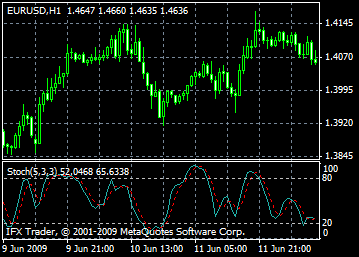# Stochastic Oscillator indicator formula and settings: description, adjustment and application

Technical Indicator Stochastic Oscillator compares current closing price with its price range for a certain time period. The Indicator is indicated as two lines. The main line is called %K. The second line, called %D, is a Moving Average of %K. The %K line is usually indicated as a firm line and the %D line is usually displayed as a dotted graph.

There are three the most popular ways to interpret a Stochastic Oscillator.

- Buy when the Stochastic Oscillator (either %K or %D) falls below a certain level (as a rule 20) and then rises above this level. Sell when the Oscillator rises above a certain level (as a rule 80) and then falls below this level;
- Buy when the %K line rises above the %D line. Sell if the %K line is below the %D line;
- Monitor divergences. For example: prices form a series of new highs and the Stochastic Oscillator is failing to surpass its previous highs.#### Calculation

The Stochastic Oscillator has four variables:
- %K periods. This is the number of time periods used in the stochastic calculation;
- %K Slowing Periods. This value controls the internal smoothing of %K. A value of 1 is considered a fast stochastic; a value of 3 is considered a slow stochastic;
- %D periods. This is the number of time periods used when calculating a moving average of %K;
- %D method. The method (i.e., Exponential, Simple, Smoothed, or Weighted) that is used to calculate %D.

The formula for %K is:
%K = (CLOSE-LOW(%K))/(HIGH(%K)-LOW(%K))*100
Where:
CLOSE - is today`s closing price;
LOW(%K) - is the lowest low in %K periods;
HIGH(%K) - is the highest high in %K periods.
The %D moving average is calculated according to the formula:
%D = SMA(%K, N)
Where:
N - is the smoothing period;
SMA - is the Simple Moving Average.

Can't speak right now?